Tl;dr An automated market maker implies that instead of a human determining sell/buy prices, they are determined by various parameters we are going to walk you through below. 🙂

Now.. let’s talk about price calculation!

# Some definitions

• Liquidity Pool: simply put, a liquidity pool is a shared pot of digital assets (i.e. tokens)

• Spot price - s0: As defined in the pool

• Delta - Δ: As defined in the pool. Will be normalized to decimal value if the pool type is exponential

• All the below values should be normalized to decimal values for calculations (10% = 0.1, 100bps = 0.01, etc.)

• Royalty percentage - r: As defined in the pool (for when the pool is buyer) or the input params (for when the pool is seller)

• Seller fee basis points - f: As defined in metadata

• Maker fee - m: Maker fee, as defined by our maker/taker fee structure

• Taker fee - t: Taker fee, as defined by our maker/taker fee structure

• Effective LP fee - l: conditional function where it is zero if the pool is one sided and taken from the pool data if the pool is two sided

• Effective price - e: What the person interacting with the pool expects to receive (if pool is buyer) or pay (if pool is seller)

With every trade that occurs, the spot price will update to better reflect the market conditions implied by a trade. After the pool buys a token, the price the pool is willing to pay for another token will be lower; similarly, after the pool sells a token, the price the pool is willing to sell another token will be higher.

In the below functions, only discuss how spot price changes with each trade, effective prices will be discussed later. We will define sn as the spot price after n trades in a specific direction. If n is positive, it will be after n buy transactions, and if n is negative, it will be after n sell transactions. The graphs shown are also made such that once n becomes negative, it will be red and between parentheses.

## Linear pools

For linear pools, the delta is interpreted as lamports. For ease of representation, we show delta and spot price in SOL

sn = s0 − nΔ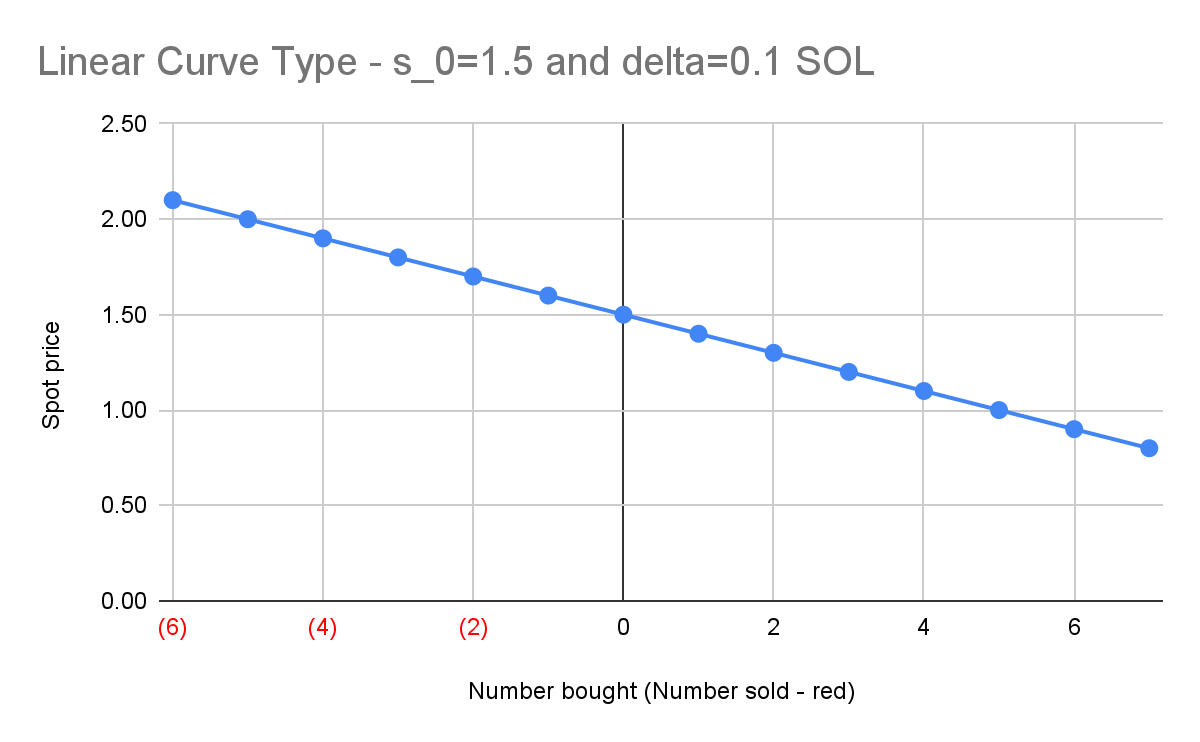## Exponential pools

For exponential pools, the delta is interpreted as basis points. For ease of representation, we show delta in percentage.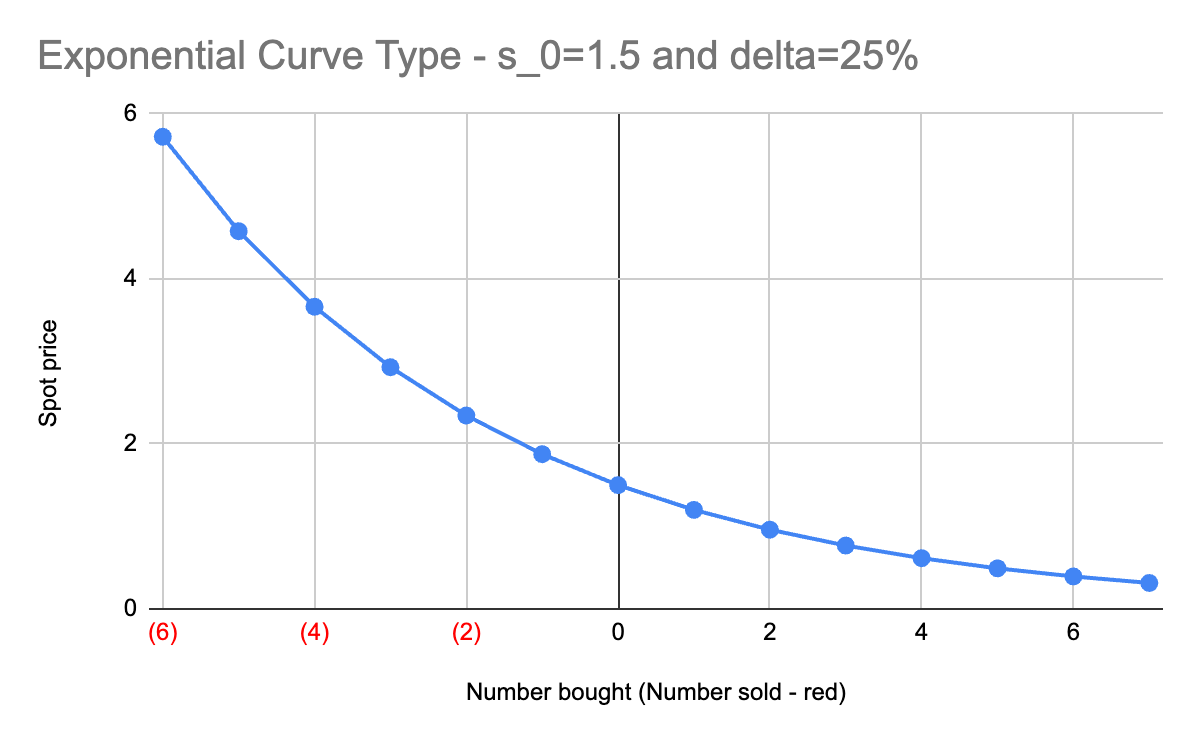# Effective Price

To enforce a spread between the buy and sell prices, we calculate effective price for selling with s−1instead of s0. However, the royalty, lp fee, and taker fee also contribute to the spread between the buy and sell prices.

Note: The pool will ignore user input r values if the token is a pNFT or has OCP enforced royalties. For pNFT, we will always enforce full royalties, and for OCP tokens, we will either enforce full royalties, or use the dynamic royalty if available.

We need to determine if a pool is two-sided to decide whether to charge LP fees. We can determine if a pool is two-sided by looking at how much SOL is deposited in the pool and how many NFTs are deposited into the pool. If the SOL amount deposited is > spot price and the amount of NFTs deposited is > 1, then it is a two-sided pool.

e = s0(1−rflt)

## Pool Selling (AKA AMM Listings)

e = s−1(1+rf+l+t)

## Example graphs

The graphs below assume that the pool is two sided.

Given a pool where the curve type is exponential, s0 = 1.5, Δ = 25%, r = 50%, f = 2%, ,t = 1.5%, l = 1%, the initial effective buy and sell prices are shown below. This shows that for example, after selling 2 tokens, the taker price to buy the 3rd taken will be around 3.03 SOL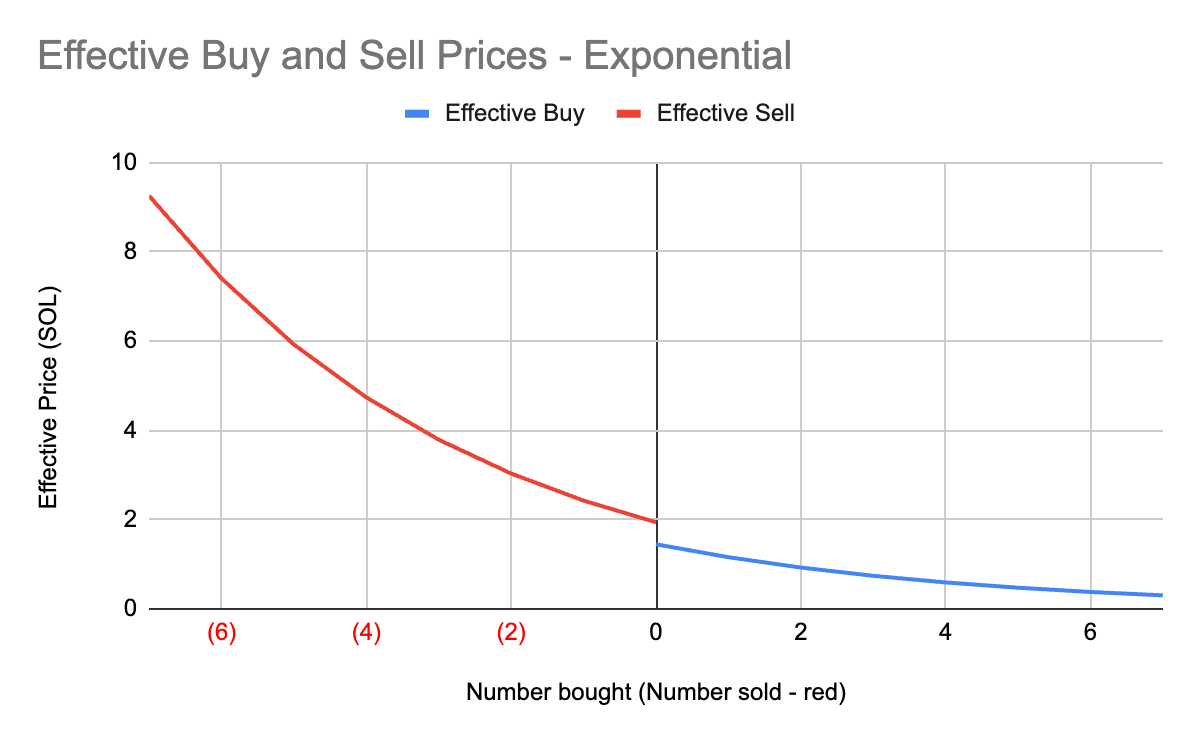For readability reasons, the red and blue lines do not extend past the 0, even though the pool can still buy NFTs after selling some. However, if the pool was to buy a token, we can simulate this by extending the lines to show what the effective buy/selling prices will be. After buying one token, the pool is willing to sell at approximately 1.5 SOL and buy at 1.15 SOL instead of the previous 1.94 SOL and 1.44 SOL.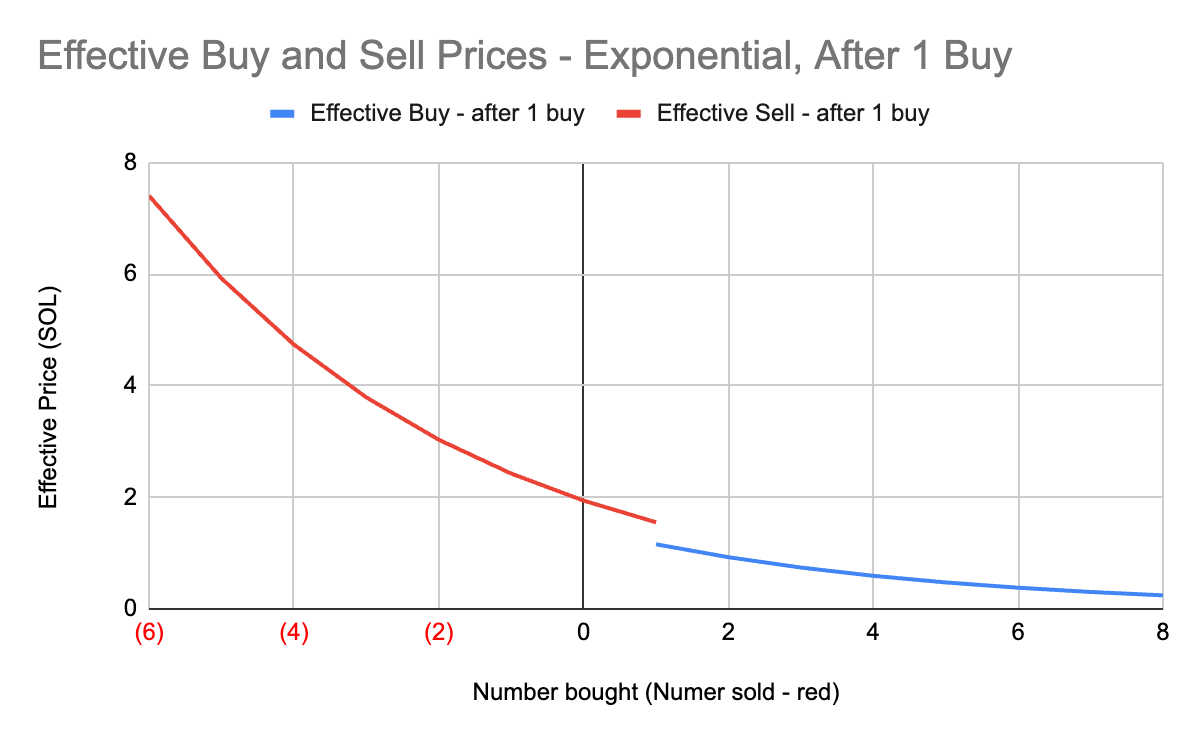Below is an example of a linear curve, with the same values as the exponential curve except for delta being 0.1 SOL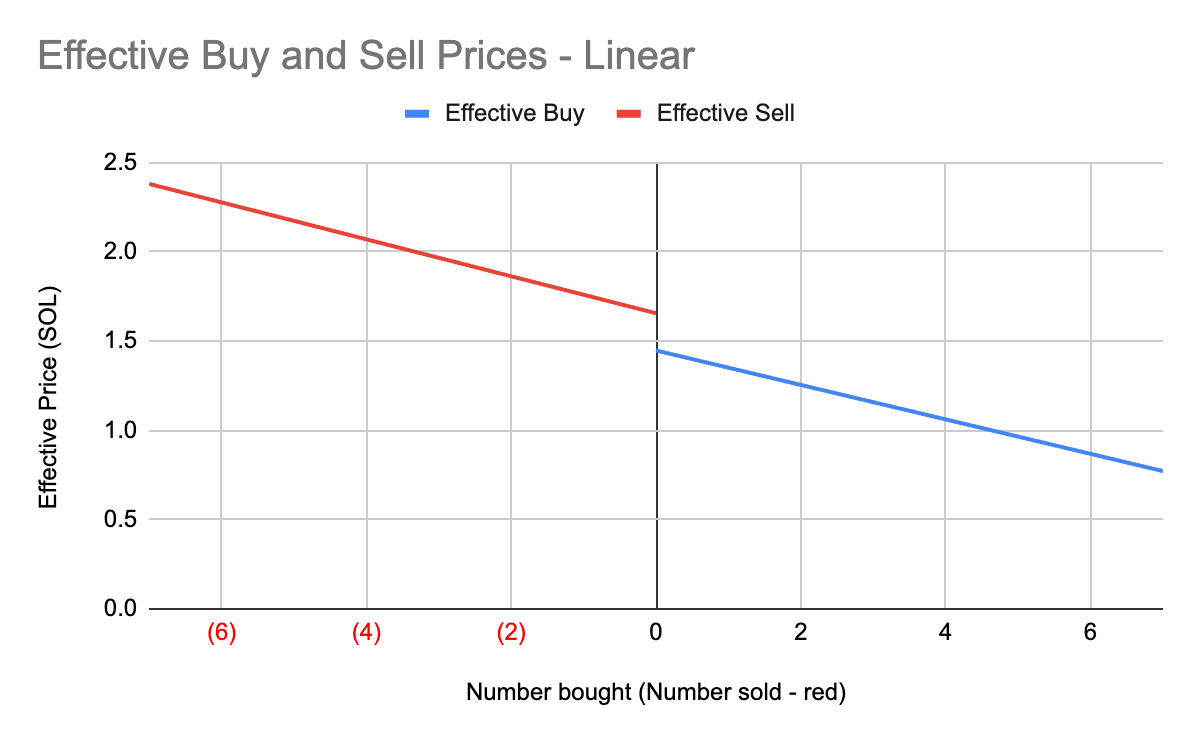To find the number of NFTs to sell, it is just the number of NFTs that are deposited into the pool. The process to find the number of NFTs buyable is a little trickier, but the easiest way to do it is probably iteratively, as seen in the code snippet below.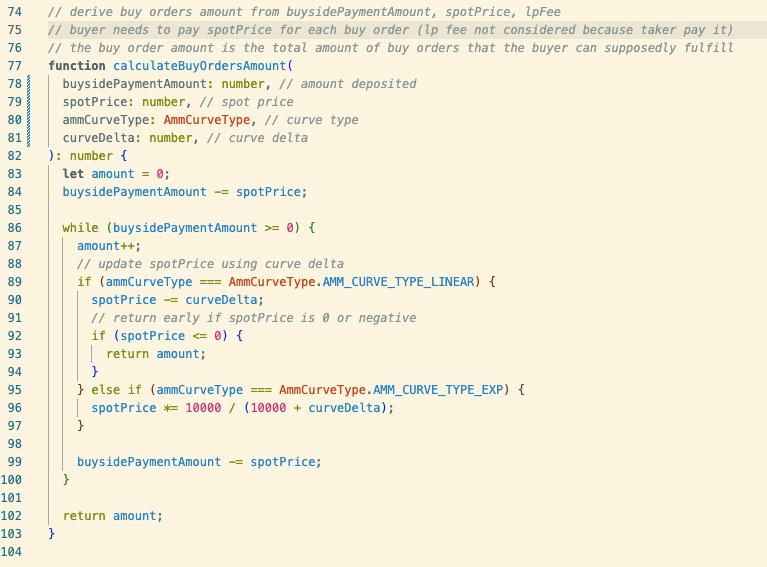To do it in a non-iterative fashion, we can use the arithmetic and geometric sequence functions to find that if n is the number of NFTs buyable given a spot price and curve delta, and d is the amount deposited, then

• For linear pools: ${n}{=}\frac{{\left(}{2}{s}{+}{\Delta }{\right)}{-}\sqrt{{\left(}{2}{s}{+}{\Delta }{{\right)}}^{{2}}{-}{8}{\Delta }{d}}}{{2}{\Delta }}$

• This is assuming that the deposited amount d is less than the amount needed to fulfill $\frac{{s}}{{\Delta }}$ buys. Otherwise, n = d

• For exponential pools: we can define , then ${n}{=}{{l}{o}{g}}_{{\gamma }}{\left(}\frac{{d}{\left(}{\gamma }{-}{1}{\right)}}{{s}}{+}{1}{\right)}$

# Maker Fees

Most of the above discussion does not involve maker fees. Adding maker fees to the current iteration of MMM will change the behavior in two ways

• Since the pool maker pays the maker fees from the amount deposited, the above calculations for NFTs buyable will change to replace s with s(1+m)

• The pool will become unsustainable. Since maker fees are not factored into the spot price, every time a trade occurs, the deposited payment will be lowered by the maker fee amount paid. This amount is not received upon the next trade on the opposite side, as what would usually happen with spot price.

• We can change this with a new iteration of MMM where LP fees, which are always paid by the taker, can be used to fund maker fees.